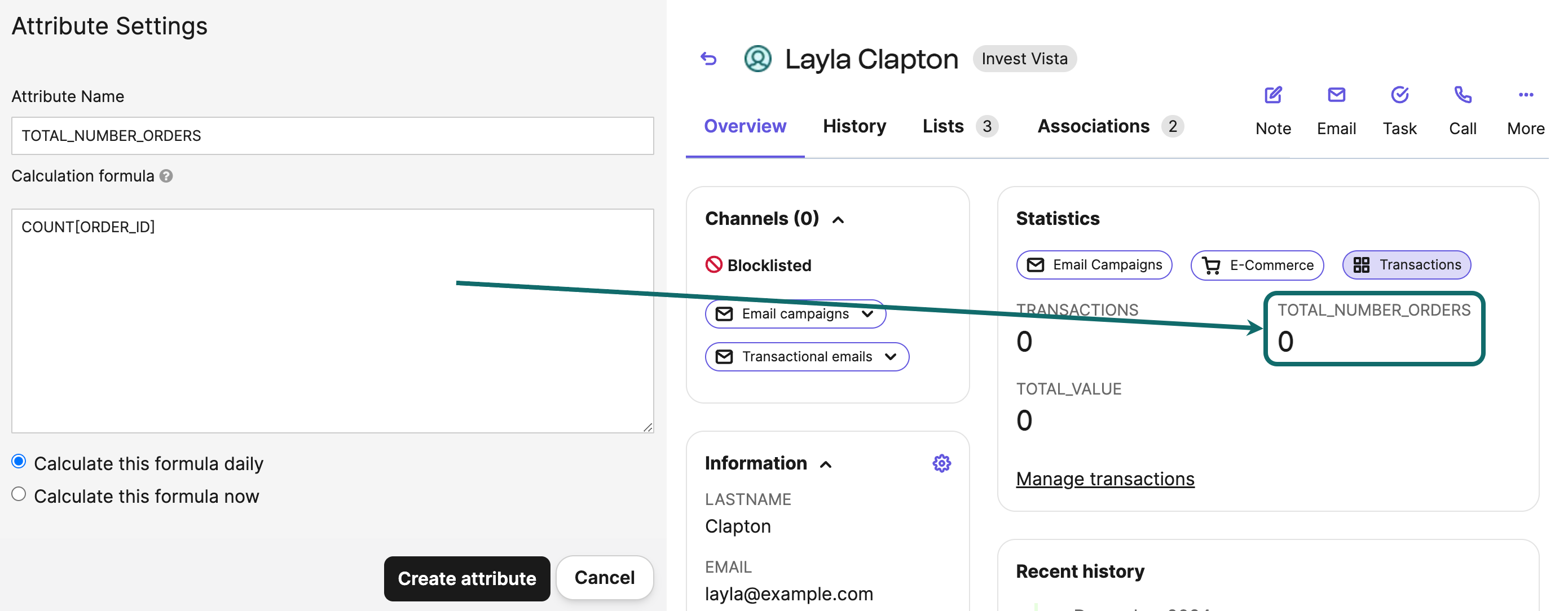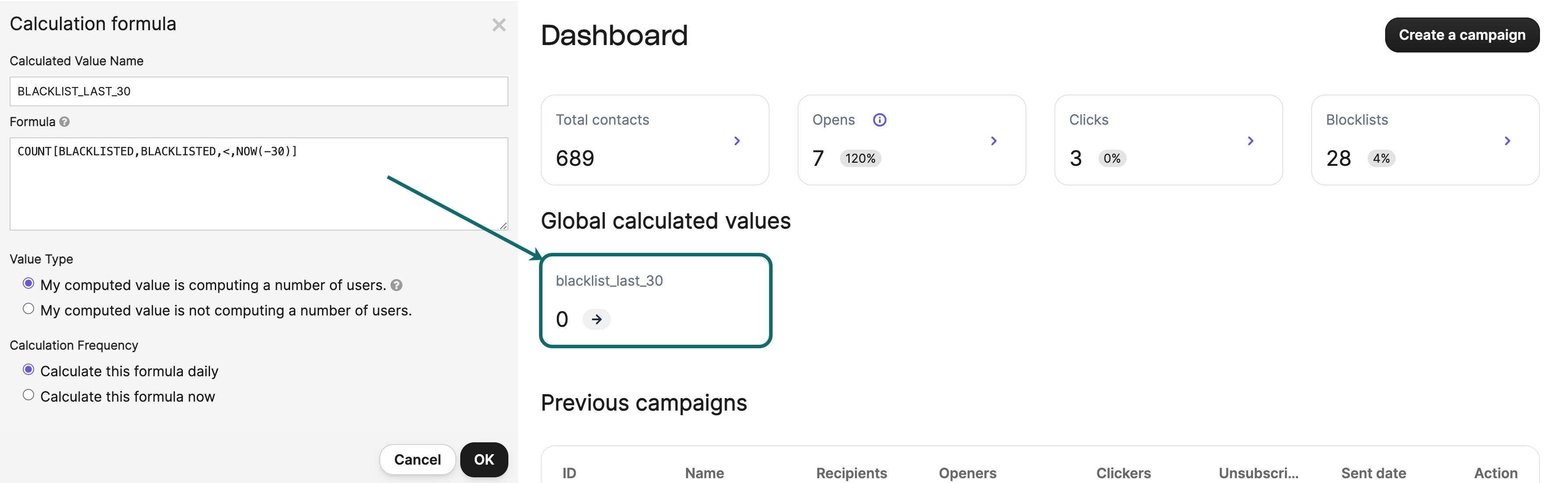# Build and use formulas

In this article, we will explain how to build and use formulas to calculate different data using contact attributes and transactional parameters.

# How to build a formula?

In Brevo, the two basic functions to build formulas are COUNT and SUM.

## COUNT - Count specific data

The COUNT function can be used to count specific data.

 COUNT[A] Will count the number of A. COUNT[A,B,C,D]A is mandatory; B, C, and D are optional. Will count the number of A, use B as a first condition, use C as an operator (=, < or >), and use D as a second condition.

## SUM - Calculate the total value of specific data

💡 Good to know
You cannot use a text attribute with a SUM function.

The SUM function can be used to calculate the total value of specific data.

 SUM[A] Will calculate the total value of A. SUM[A,B,C,D]A is mandatory; B, C, and D are optional. D can be a fixed number or can be used with the command NOW where NOW(-x) is the date from x days ago. Will calculate the total value of A, use B as a first condition, use C as an operator (=, < or >), and use D as a second condition.

# How to use formulas?

## Calculated values

A formula can be used in a calculated value to calculate specific data for each of your contacts using transactional parameters. Calculated values are displayed on your contacts' details page.

To learn how to add calculated values, check our dedicated article What are the different types of contact attributes?.➡️  For example
If you are using the transactional parameter ORDER_ID to store orders, ORDER_VALUE to store the total amount of orders, and ORDER_DATE to store the order date:
• COUNT[ORDER_ID]: count the total number of orders made by a contact.
• COUNT[ORDER_ID,ORDER_VALUE,>,50]: count the total number of orders over 50€ made by a contact.
• SUM[ORDER_VALUE]: calculate the total value of orders made by a contact.
• SUM[ORDER_VALUE,ORDER_DATE,>,NOW(-30)]: calculate the total value of orders made in the past 30 days by a contact.

## Global calculated values

A formula can be used in a global calculated value to calculate custom statistics for your contact lists and emails using contact attributes. Global calculated values are displayed under the Global Computed Values section of your dashboard.

To learn how to add a global calculated value, check our dedicated article Can I add custom statistics to calculate?.➡️  For example
• COUNT[BLACKLISTED,BLACKLISTED,<,NOW()]: count all blocklisted contacts until now.
• COUNT[BOUNCES,SOFT,<,NOW(-30)]: count all soft bounces in the last 30 days.
• COUNT[BOUNCES,HARD,<,NOW()]: count all hard bounces until now.